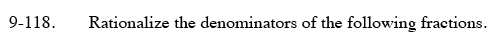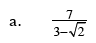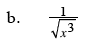### Home > PCT > Chapter Ch9 > Lesson 9.3.2 > Problem9-118

9-118.Multiply the numerator and denominator by the conjugate.

$\left(\frac{7}{3-\sqrt{2}}\right)\left(\frac{3+\sqrt{2}}{3+\sqrt{2}}\right)$

$\frac{7\left(3+\sqrt{2}\right)}{9-\sqrt{4}}$

$3+\sqrt{2}$Multiply the numerator and denominator by √ x .